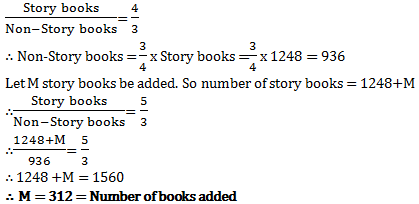# Aptitude test for MCA interview - Set 4

1)   Find the ratio of swimming speed of Raj in still water to speed of river, if ratio of time taken to go 10km upstream to time taken to go 10km downstream is 11:5?

a. 11:5
b. 4:2
c. 8:3
d. 6:5
 Answer  Explanation ANSWER: 8:3 Explanation: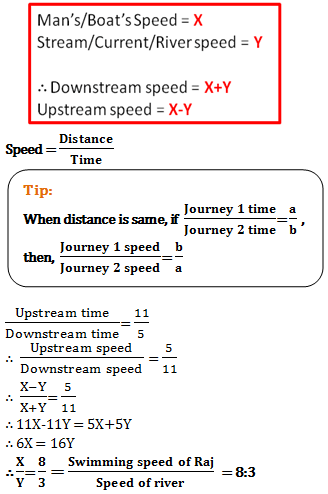2)   A has two grandsons P and Q. 13 year old P gets some money from A’s wealth and 14 year old Q gets rest of the money. But P and Q will get money only when they turn 25 years old. Till then the money is in a bank getting interest at rate 4% compounded annually. When both turn 25, they receive the same amount. How much had A given Q initially, if total money with A was Rs.25500?

a. Rs. 12500
b. Rs. 13000
c. Rs. 15000
d. Rs. 11500
 Answer  Explanation ANSWER: Rs. 13000 Explanation: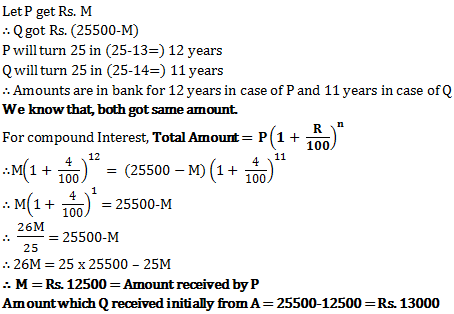3)   What will be difference in population 3 years ago and 2 years ago of Devon village, whose current population is 100000 and which is increasing at a rate of 25% every year?

a. 15250
b. 13900
c. 16400
d. 12800
 Answer  Explanation ANSWER: 12800 Explanation: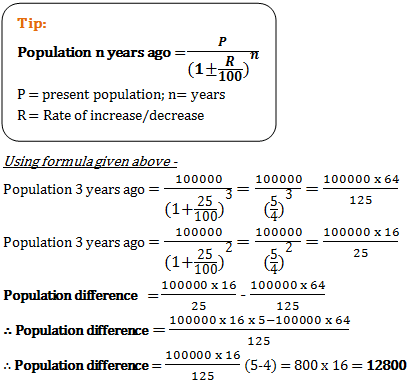4)   A milkman had water and milk mixture in a can with water to milk ratio 5:7. He accidently spills 9 liters of the mixture. He then fills the can with water equal in quantity to spilled mixture. This makes the water to milk ratio 9:7. How much milk did the can initially have?

a. 21 liters
b. 24 liters
c. 16 liters
d. 20 liters
 Answer  Explanation ANSWER: 21 liters Explanation: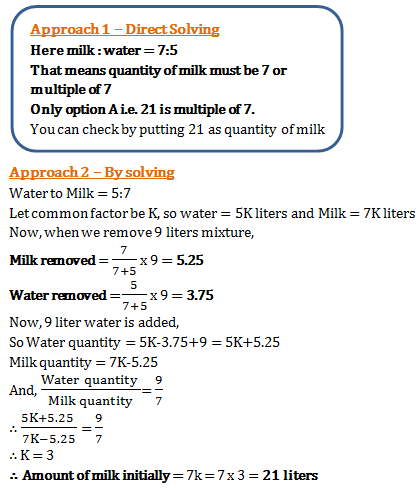5)   Chaman has two big cans of wine and water mixture. Chaman mixes the contents of both the cans in a big container. The new mixture has half water and half wine. In what quantity did Chaman mix contents of Can 1 and 2 if Can 2 has wine to water ratio of 2:3 and Can 1 has wine to water ratio 5:3?

a. 5:3
b. 4:5
c. 5:4
d. 2:5
 Answer  Explanation ANSWER: 4:5 Explanation: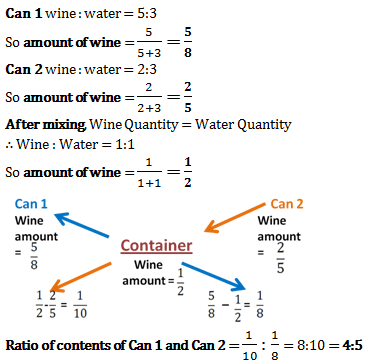6)   Speed of river is 6 km/hr. Speed of a motorboat in still water is 30km/hr. How much distance can it cover downstream in 24minutes?

a. 9.8 km
b. 864 m
c. 12.8 km
d. 14.4 km
 Answer  Explanation ANSWER: 14.4 km Explanation: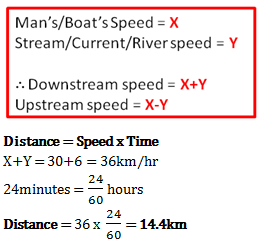7)   Rajesh has a container which has a mixture of wine and water in it. Wine and water are in the ratio 4:1. Rajesh spills some of the mixture by accident. He then replaces the spilled amount with water of same quantity. But now the wine to water ratio became 3:2. How much water did Rajesh add?

a. 3/5
b. 1/2
c. 1/4
d. 2/7
 Answer  Explanation ANSWER: 1/4 Explanation: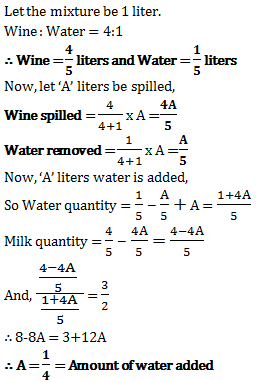8)   Gurpreet went to a shop and bought a sofa. She got a 20% discount on it. Had she gotten 25% discount she would have saved Rs. 1000 more. How much did she pay for the sofa?

a. Rs. 5000
b. Rs. 10,000
c. Rs. 20,000
d. Rs. 25000
 Answer  Explanation ANSWER: Rs. 20,000 Explanation: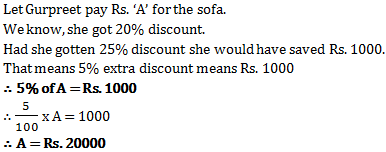9)   P and Q work together and get a payment of Rs. 1400. P can complete the work alone in just 30 days. But Q takes 40 days to complete the work alone. What is P’s share out of the wages they get?

a. Rs. 800
b. Rs. 600
c. Rs. 1000
d. Rs. 700
 Answer  Explanation ANSWER: Rs. 800 Explanation: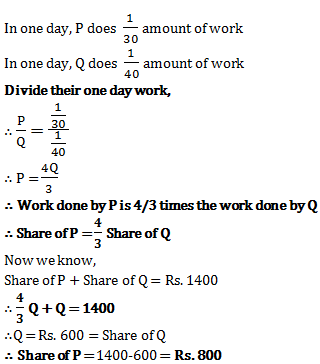10)   P and Q work together on a task for 5 days. They then leave and rest of the task is done by R in just 2 days. All the three together are paid Rs. 450. What will be R’s share out of the payment if P alone could do the task in 10 days and Q alone can do the task alone in 15 days?

a. Rs. 100
b. Rs. 75
c. Rs. 225
d. Rs. 50
 Answer  Explanation ANSWER: Rs. 75 Explanation: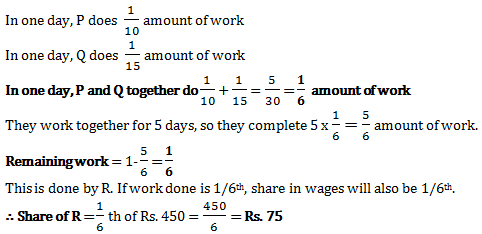11)   What will be share of Rajesh, if together Ramesh and Suresh complete only 7/11 of the task, and all three had been given the contract to finish the task for Rs. 1100?

a. Rs. 350(4/11)
b. Rs. 200
c. Rs. 400
d. Rs. 650
 Answer  Explanation ANSWER: Rs. 400 Explanation: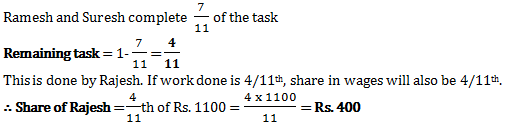12)   P and Q together earn Rs. 188 per day. Q and R together earn Rs. 152 per day. P, Q and R when working together earn Rs. 300 per day. How much does Q earn daily?

a. Rs. 43
b. Rs. 56
c. Rs. 45
d. Rs. 40
 Answer  Explanation ANSWER: Rs. 40 Explanation: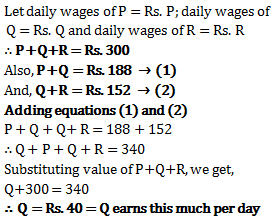13)   Ram and Shyam have been given a task of painting a house for Rs. 800. With the help of Rita, they complete the job in just 3 days. Had Ram alone be doing the task, he would need 6 days. If Shyam alone would be doing the task, he would need 8 days. How much money will Rita get?

a. Rs. 80
b. Rs. 150
c. Rs. 100
d. Rs. 125
 Answer  Explanation ANSWER: Rs. 100 Explanation: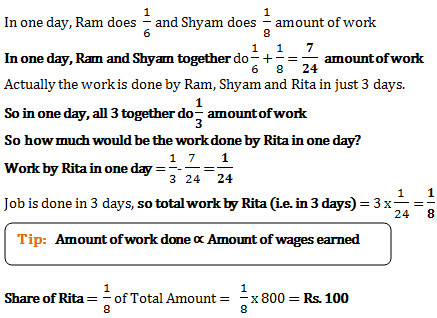14)   Raj got a new chair for 35% discount. Had Raj got no discount, Raj would have had to pay Rs. 224 more. How much did Raj pay for the chair?

a. Rs. 416
b. Rs. 640
c. Rs. 208
d. Rs. 224
 Answer  Explanation ANSWER: Rs. 416 Explanation: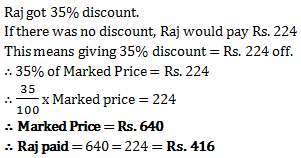15)   Raju invested Rs. 77500 in ICICI bank. In two years how much compound interest will he get, if the first year rate of interest was 10% and second year had 2% more than first year?

a. Rs. 17850
b. Rs. 17980
c. Rs. 18963
d. Rs. 16880
 Answer  Explanation ANSWER: Rs. 17980 Explanation: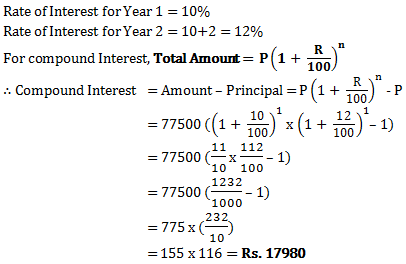16)   What is value of 945.341-1042.792+875.435+31.025?

a. 908.004
b. 810.008
c. 795.659
d. 809.009
 Answer  Explanation ANSWER: 809.009 Explanation: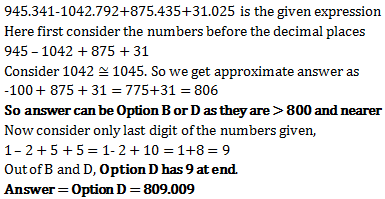17)   What will be ratio of simple to compound interest on two same sums invested in SBI at rate of interest of 8% kept for 3 years?

a. 1875/2029
b. 1/2.5
c. 1903/2156
d. 4/9
 Answer  Explanation ANSWER: 1875/2029 Explanation: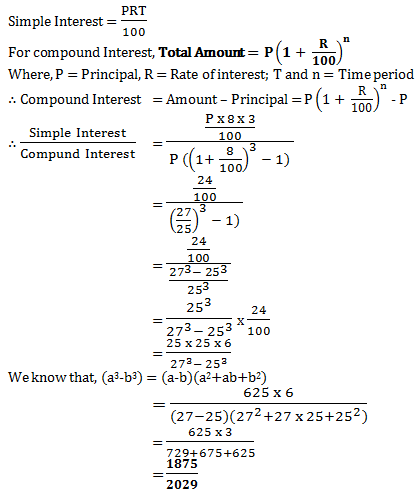18)   In the India-Australia one day match, due to rain, India needed 324 runs in 48 overs to win. In initial 10 overs, the average scoring rate was 6, but in next 10 overs it increased to 8.5. It then declined to 5.5 in next 10 overs and again rose to 7 in next 10 overs. To win the match now what average is needed?

a. 8.25
b. 6.75
c. 7.75
d. 7.0
 Answer  Explanation ANSWER: 6.75 Explanation: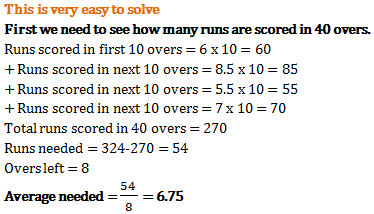19)   A man goes to Mumbai from Pune at a speed of 4 km/hr and returns to Pune at speed of 6km/hr. What is his average speed of the entire journey?

a. 4.8km/hr
b. 5 km/hr
c. 4.2 km/hr
d. 5.6 km/hr
 Answer  Explanation ANSWER: 4.8km/hr Explanation: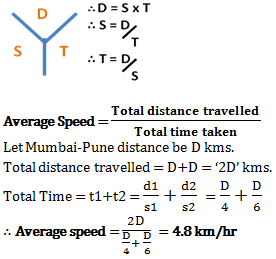20)   In a library, the ratio of number of story books to that of non-story books was 4:3 and total number of story books was 1248. When some more story books were bought, the ratio became 5:3. Find the number of story books bought.

a. 312
b. 321
c. 936
d. 1560
 Answer  Explanation ANSWER: 312 Explanation: